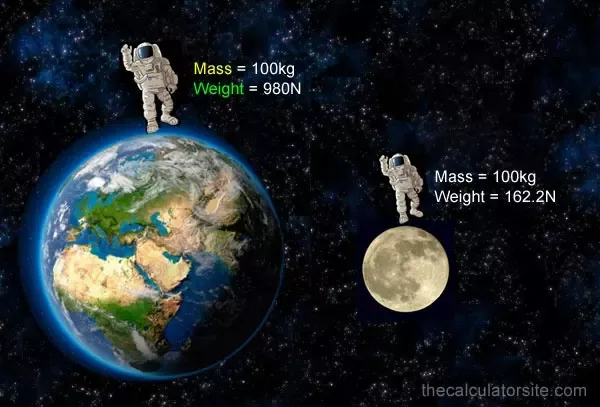# Weight Study Guide

INTRODUCTION

Have you ever tried and failed to lose weight? Don’t be depressed; you just might be on the wrong planet! Technically, the weight of an object varies depending on its location. If you choose to go to the moon to test this idea, you’ll find that in just a few hours, you’ll have lost two-thirds of your weight. Look out, fat shamers!Source

## WHAT IS MASS?

• The meaning of mass is that it’s the quantity of matter that makes up a thing.
• Mass remains constant regardless of where you are at any particular time.
• Mass is indestructible and can never be zero.
• This means that everything in this universe has mass if it exists.
• The metric system measures the mass in kilograms and grams. Now, let’s talk about weight.

## WHAT IS WEIGHT?

• The meaning of weight is that it is the measurement of the force of gravity acting on a body.
• The weight of an object fluctuates depending on where it is, and it is a vector (a quantity fully characterized by both a magnitude and a direction) with a pull towards the center of the earth you’re standing on.

The weight formula is as follows:

W = mg

Where ‘w’ is weight and ‘m’ is the mass of the object, and ‘g’ is the acceleration due to gravity, which is 9.8 m/s2.

Because weight is a force, it has the same SI unit as a force. The SI unit for weight is Newton (N).

## RELATION BETWEEN WEIGHT AND MASS

Newton’s law theory states that a free-falling object has an acceleration “g” as the magnitude may be used to deduce the relationship between weight and mass.

If a 1-kilogram item descends with an acceleration due to gravity (a) of 9.8 m.s-2, the magnitude of the force can be calculated using F = ma. Therefore, the value of F here would be 9.8 newtons. It may be deduced from the weight-mass relationship that an object with a mass of 1kg will have a weight of 9.8N.

## SUMMARY

• A substance’s mass is the amount of matter it contains.
• The net force on a falling object, or its gravitational force, is its weight.
• W = mg is the formula used to calculate the weight.

## FAQs

1. Is weight in kg or N?

Weight is a force and therefore is calculated in newtons.

2. Is mass or weight in Newtons?

Weight being a force is calculated in Newtons.

3. Is weight and mass the same?

No, mass is the amount of matter that every existing object contains and is constant, whereas weight is a force that is exerted on an object and can be variable.

We hope you enjoyed studying this lesson and learned something cool about Weight! Join our Discord community to get any questions you may have answered and to engage with other students just like you! We promise, it makes studying much more fun!😎

]]>